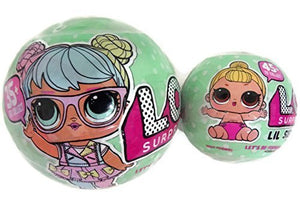# Lol Surprise Doll Series 2 Bundle Of Lets Be Friends! And Her Lil Sister

L.O.L. Surprise!

• \$40.16
Unit price per
• Save \$24.75

• L

• O

• L

• S

• u

• r

• p

• r

• i

• s

• e

• D

• o

• l

• l

• S

• e

• r

• i

• e

• s

• 2

• B

• u

• n

• d

• l

• e

• i

• n

• c

• l

• u

• d

• e

• s

• (

• 1

• )

• L

• e

• t

• s

• B

• e

• F

• r

• i

• e

• n

• d

• s

• !

• a

• n

• d

• (

• 1

• )

• H

• e

• r

• L

• i

• l

• S

• i

• s

• t

• e

• r

LOL Surprise Doll Series 2 Bundle includes (1) Lets Be Friends! and (1) Her Lil Sister